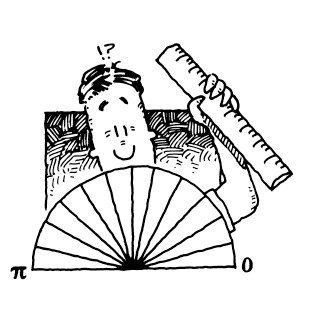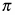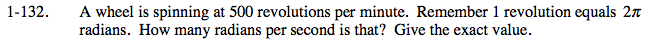### Home > PC > Chapter 1 > Lesson 1.4.1 > Problem1-132

1-132.A wheel is spinning at 500 revolutions per minute. Remember 1 revolution equals 2radians. How many radians per second is that? Give the exact value. Homework Help ✎First, calculate the number of Radians per minute.

Second, divide by 60 to calculate the number of Radians per second.

$\frac{50\pi}{3}$